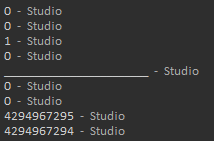# Help with bit shifting

Disclaimer: I am quite new to working with the bit32 library and bit shifting in general. Bear with me as I attempt to explain what I did.

I’m experimenting with the bit32 library and made a few observations about how it works in comparison to JavaScript’s shift operators.

## Lua

Code

``````print(bit32.rshift(0, 0))
print(bit32.rshift(0, 1))
print(bit32.rshift(1, 0))
print(bit32.rshift(1, 1))
print("________________________")
print(bit32.rshift(0, 0))
print(bit32.rshift(0, -1))
print(bit32.rshift(-1, 0))
print(bit32.rshift(-1, -1))
``````

Output## JavaScript

1. It appears the bit32 library uses the no-sign shift operator (>>> instead of >>). How could I use the >> equivalent in Luau?
2. In the final example, Lua returns `4294967294` for `bit32.rshift(-1, -1)` but JS returns `1` for the same equation. Why (JS overflow?)?
1 Like

It looks like lua fills vacant bits with `0` regardless, whereas JavaScript will fill vacant bits with the sign bit (something to do with two’s complement, I’m not very sure)

1 Like

I think the best solution for this is to use `bit32.replace(shiftedNum, -1, 31, 31 - shiftAmount)` when `num < 0`.

2 Likes

So for this example:

``````-- Lua
bit32.rshift(-1, 0) -- 4294967295

-- JS
-1 >> 0 -- -1
-1 >>> 0 -- 4294967295
``````

Using

``````bit32.replace(bit32.rshift(-1, 0), -1, 31, 31 - -1)
``````

and

``````bit32.replace(bit32.rshift(-1, 0), -1, 31, 31 - 0)
``````

yield a “trying to access non-existent bits” error.

1 Like

Maybe I read the documentation wrong, perhaps you need to use a loop instead, but the base premise is to replace bits with a different bit so that a negative number is bit-shifted with 1s instead of 0s

1 Like

turns out lua just decides that the integer becomes unsigned or something, but this code snippet seems to do the trick:

``````local function rshiftsigned(num: number, shiftAmount: number)
local shiftedNum = bit32.rshift(num, shiftAmount)

if num < 0 then
return shiftedNum - 2 ^ 32
else
return shiftedNum
end
end
``````
2 Likes

The `bit32` library’s functions are only compatible with signed 32 bit integers.
https://www.lua.org/manual/5.2/manual.html#6.7

Unless otherwise stated, all functions accept numeric arguments in the range (-251,+251)

1 Like

You can do that with ro-typescript for question 1. For 2 it shows that the bit32 library will absolute your input causes your binary thing looks like this 10000000 and when you right shift it in lua you get essentially a really high number (2^32-1). For javascript, it’s probably cause it uses left shift if the input is negative cause it makes no sense to shift bits by a negative number. right shift and left shift actually just execute a couple bitmasks and AND operations

2 Likes

To make this work as a left shift, would all you need to do is change bit32.rshift to bit32.lshift?

you should just try it and see

2 Likes
``````local function alshift(x: number, disp: number): number
local y = bit32.lshift(x, disp)

if y >= 2 ^ 31 then
return y - 2 ^ 32
end

return y
end

local function arshift(x: number, disp: number): number
local y = bit32.rshift(x, disp)

if y >= 2 ^ 15 then
return y - 2 ^ 16
end

return y
end
``````